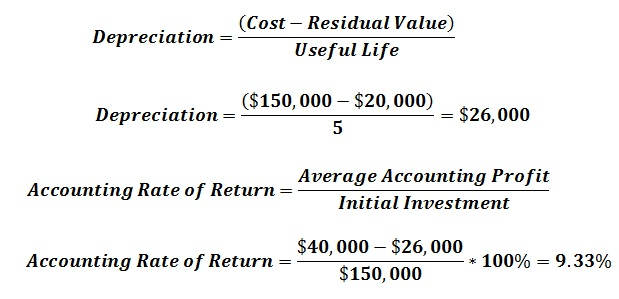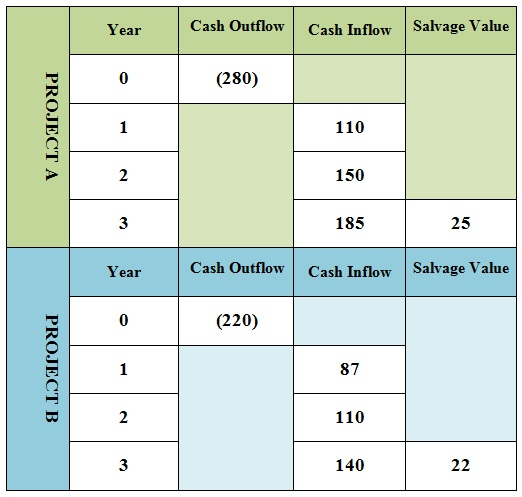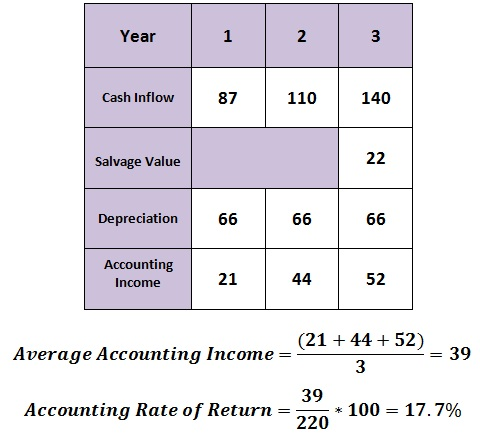# How to Calculate Accounting Rate of Return

Knowing how to calculate Accounting Rate of Return (ARR) is important in capital budgeting as it is used to determine the appropriateness of a particular investment. When the answer for ARR exceeds a specific rate, which is accepted by the company, then the project will be selected.

## Formula to Calculate Accounting Rate of Return (ARR)

ARR can be calculated using the below formula:Average Accounting profit is the mean of the accounting income that is expected to earn within the lifetime of the project. Instead of initial investment there are instances where the average investment is used.

The decision to accept or reject a project is based on the value generated in ARR. The project is accepted if the ARR value is equal to or greater than the required rate of return. In mutually exclusive projects, the project that generates the higher ARR is accepted.

## Examples to calculate accounting rate of return (ARR)

Following examples illustrate the ways of calculating ARR.

E.g.1: Project A is having an initial investment of \$150,000, and it is expecting to generate an annual cash inflow of \$40,000 for 5 years. Depreciation is calculated based on the straight line method. The scrap value is about \$20,000 at end of the 5th year.
ARR can be calculated as,E.g.2: Compare the following mutually exclusive projects based on ARR and identify which project is financially feasible to undertake.Project ASince ARR of a project A is higher that of B, it is more favorable than the project B.